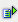The function odd (num) returns the next higher odd whole-numbered value. Negative values are rounded from 0 away.

Syntax: =odd(num)Example =odd(2.2) is 3 =odd(-2,2) is -3